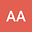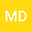loading page

A numerical method for finding solution of the distributed order time-fractional forced Korteweg-de Vries equation including the Caputo fractional derivative
••• A. Aminataei,
• mohammadhossein derakhshan
A. Aminataei
K.N. Toosi University of Technology
Author Profilemohammadhossein derakhshan
K.N.Toosi University of technology
Author Profile## Abstract

In this paper, for the first time, the distributed order time-fractional forced Korteweg-de Vries equation is studied. We use a numerical method based on the shifted Legendre operational matrix of distributed order fractional derivative with Tau method to find approximate solution of distributed order forced Korteweg-de Vries equation. This shifted Legendre operational matrix of distributed order fractional derivative with Tau method are used to reduce the solution of the distributed order time-fractional forced Korteweg-de Vries equations to a system of algebraic equations. An error analysis and convergence are obtained. Finally, to display the applicability and validity of the numerical method some examples are implemented.

#### Peer review status:UNDER REVIEW

20 Aug 2020Submitted to Mathematical Methods in the Applied Sciences
24 Aug 2020Assigned to Editor
24 Aug 2020Submission Checks Completed
31 Dec 2020Reviewer(s) Assigned